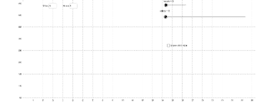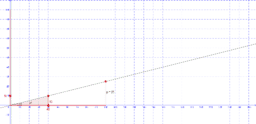# first book

Author:
welllia
The slope of any line passing through (0, 0) can be seen as y/x. Any part/whole fraction can be represented as a slope ratio with the numerator = y and the denominator = x. Use the slider on the x-axis to determine the whole, and the slider on the y-axis to represent the part in a fraction (i.e., y/x). Similarly, a ratio of a: b can be represented as a/b using the slider on the y-axis for a and the slider on the x-axis for b. The ratio of y/x is proportional to the percent value (i.e., p/100). So, for example, a ratio of 10/40 can be seen as equivalent to 25/100, which is 25%. The ratio of the unit rate (i.e., r/1) is also proportional to any ratio expressed as y/x. So, a ratio of 10/40 has a unit rate of 1/4: 1, or 0.25.
•### Rates: miles per hour

•### Graph the Line

•